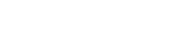# math.cos

`FLOATmath.cosFLOATx`

Available inall subroutines.

Computes the cosine of its argument `x`, measured in radians.

## Parameters

`x` - Floating point value representing an angle in radians.

## Return Value

Upon successful completion, this function returns the cosine of `x`.

If `x` is `math.NAN`, a NaN will be returned.

If `x` is ±0, the value 1.0 will be returned.

If `x` is `math.POS_INFINITY` or `math.NEG_INFINITY`, a domain error occurs and a NaN will be returned.

## Errors

If the `x` argument is `math.POS_INFINITY` or `math.NEG_INFINITY`, then `fastly.error` will be set to `EDOM`.

## Example

``````declare local var.fo FLOAT;
set var.fo = math.cos(math.PI_2);``````

BETA